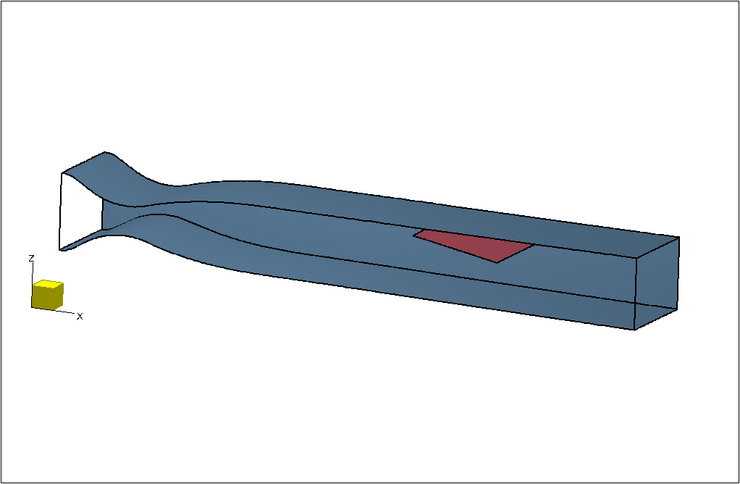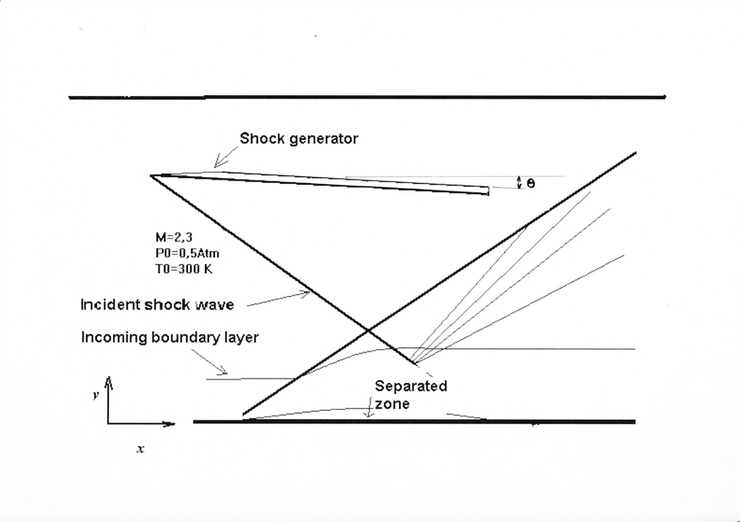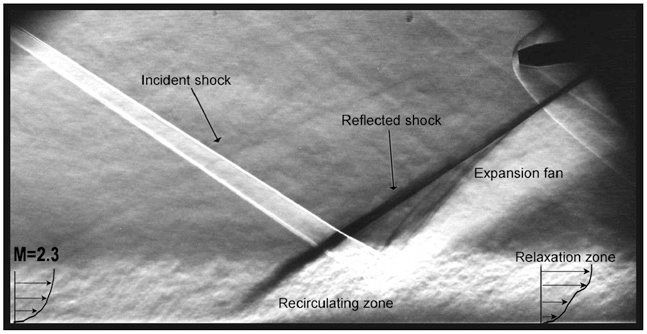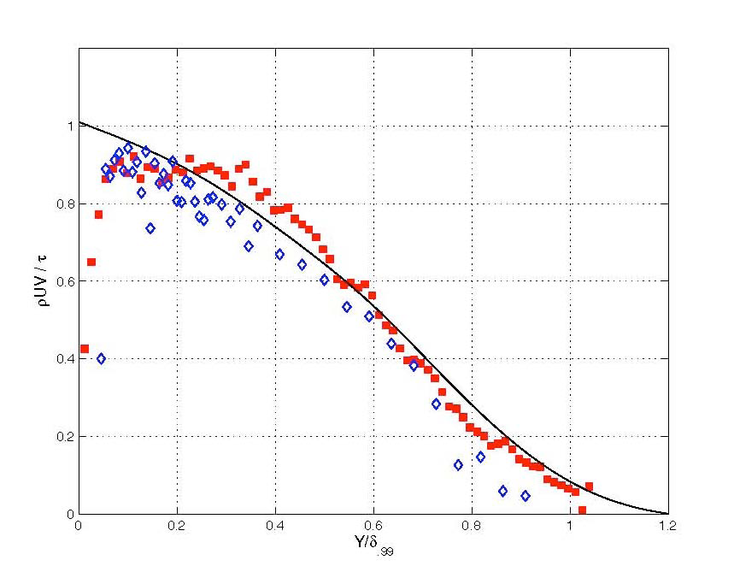# Test Case Study

## Brief Description of the Study Test Case

The flow under investigation is an oblique shock reflection on a flat plate where a turbulent boundary layer is formed (see Figure 2). In this case, the flat plate is the floor of the test section. The shock wave is produced by a shock generator. The angle ${\left.\theta \right.}$of the shock generator with respect to the external flow is supposed to be the same as the flow deflection; this is a very good approximation in the present flow conditions. Two cases are studied, corresponding to flow deflections ${\left.\theta \right.}$of 8° and of 9.5° at the nominal Mach number 2.25. They are both separated. This experiment is designed to provide the characteristics of the low frequency unsteadiness found in such conditions, and affecting the reflected (or separation) shock wave and the separated zone itself. The deflection is produced by a shock generator, i.e. a tilted flat plate, fixed on the ceiling of the wind tunnel, and leaving a sufficient gap to let a passage to the ceiling boundary layer, without affecting the flow around the shock generator leading edge. The implementation of the shock generator is given in Figure 1.Figure 1: Sketch of the test section showing the contoured nozzle block and the shock generator

A sketch of the configuration is given in Figure 2.Figure 2: Sketch of the interaction (not to scale)

The external Mach number, i.e. in the external flow upstream of the interaction is ${\left.M_{e}=2.25\right.}$, stagnation pressure in the potential flow upstream of the interaction is ${p_{\mathit {tref}}=50663\ \mathrm {N/m^{2}} }$; the stagnation temperature in the outer flow ${\left.T_{\mathit {tref}}\right.}$is typically atmospheric, and remains close to 300K. The incoming boundary layer is fully turbulent. It develops on a flat plate with nearly adiabatic constant wall temperature. The conditions in the incoming boundary layer are summed up in the following tables.

The first campaign of measurements called "case-2006" was performed with the following parameters:

 ${x\ (\mathrm {mm} )}$${\left.M_{e}\right.}$${p_{\mathit {tref}}\ (\mathrm {N/m^{2}} )}$${T_{\mathit {tref}}\ (\mathrm {K} )}$${\left.\delta \ (99\%,\ \mathrm {mm} )\right.}$${\left.R_{\delta }\right.}$${\left.\theta \ (\mathrm {mm} )\right.}$${\left.R_{\theta }\right.}$${\left.260\right.}$${\left.2.25\right.}$${\left.50663\right.}$${\left.300\right.}$${\left.11.0\right.}$${\left.58760\right.}$${\left.0.95\right.}$${\left.5070\right.}$The origin of the abscissa is taken at the end of the contoured part of the nozzle block. ${\left.R_{\delta }\right.}$and ${\left.R_{\theta }\right.}$are respectively the Reynolds numbers based on layer thickness and on momentum thickness. A second campaign called "case-2007" corresponds to the following conditions, in which the thickness of the incoming boundary layer is smaller than in the previous case.

 ${x\ (\mathrm {mm} )}$${\left.M_{e}\right.}$${p_{\mathit {tref}}\ (\mathrm {N/m^{2}} )}$${T_{\mathit {tref}}\ (\mathrm {K} )}$${\left.\delta \ (99\%,\ \mathrm {mm} )\right.}$${\left.R_{\delta }\right.}$${\left.\theta \ (\mathrm {mm} )\right.}$${\left.R_{\theta }\right.}$${\left.260\right.}$${\left.2.25\right.}$${\left.50663\right.}$${\left.300\right.}$${\left.10.0\right.}$${\left.53420\right.}$${\left.0.87\right.}$${\left.4640\right.}$The details of the geometry and of the flow conditions can be accessed in the UFAST data base in Doerffer (2009). A description of the geometry and the CAD files are given here:

The measured quantities are the wall pressure (mean, rms value and spectra) along the interaction, and 2-d velocity fields in vertical planes obtained by Particle Image Velocimetry (PIV) and by Laser Doppler Velocimetry (LDV). The two components (longitudinal, normal to the wall) ${\left.(U,V)\right.}$of the mean velocity and ${\left.(u',v')\right.}$of the fluctuating velocity have been measured. The Reynolds stresses ${{\overline {u'^{2}}},\ {\overline {v'^{2}}},\ {\overline {u'v'}}}$have been determined. The spectra of the wall pressure, which are very sensitive to the shock system unsteadiness, have also been determined. More precisely, two measurement campaigns are given denoted by 2006 and 2007, at slightly different Reynolds number (see here above). In the 2006 campaign, wall pressure measurements were performed: fluctuations are characterized by their variance and their power spectral density. PIV measurements were performed in the symmetry plane of the test section (mean velocity and normal shear stresses). In the 2007 data, only PIV data were obtained, with Reynolds stresses including shear stresses. Finally stereo PIV was use to take measurements in horizontal planes to detect 3-d features. Four horizontal planes were considered. Mean velocity was measured, and only in one plane (elevation h2) the normal stresses were obtained. The details are given in the 'readme' files below and the measurement results in the associated data files.

## Test Case Experiments

The experiment was carried out in the hypo-turbulent supersonic wind tunnel at IUSTI. It is a continuous facility with a closed-loop circuit. It can be operated for 4 hours with well controlled operating conditions. It is operated at a nominal Mach number of 2.25. The air is dried and the perturbations produced by the machinery are damped by appropriate devices: a Helmholtz resonator to remove the frequencies related to the compressor rotation, a heat exchanger, a drier acting continuously and a settling chamber. A regulation system stabilizes the stagnation pressure to a prescribed setting. Typically, when the wind tunnel is operated at a stagnation pressure of 0.5 bar, its variations in time are less than ±0.2%. The stagnation temperature is typically atmospheric; with a typical drift of 1K/hour. The level of background turbulence in the outer flow is essentially due to aerodynamic noise radiated by the boundary layers. Its level is less than 0.1% for velocity turbulence intensity. The range of Reynolds numbers produced in this facility encompasses moderate values (typically ${R_{\theta }\approx 5000}$); this makes the experiments well adapted to advanced modelling techniques like LES, which, in their present state are feasible for moderate Reynolds numbers.

The two-dimensional supersonic equilibrium turbulent boundary layer under investigation develops on the wind tunnel floor, which is a flat plate. The incoming conditions (inlet conditions for the interaction) are located at a distance larger than 25 cm downstream of the contoured part of the nozzle block (more than 25 boundary layer thicknesses). All x coordinates in the following correspond to an origin taken at the beginning of the flat part of the nozzle wall, and located 388.6 mm downstream of the sonic neck. Downstream of the interaction a diverging diffuser brings the flow from supersonic to subsonic conditions. Various devices are placed in the loop to prevent the propagation of aerodynamic noise.

The incoming boundary layer is turbulent and fully developed. It is subjected to a shock wave produced by a shock generator placed in the external flow, as indicated in figures 1 and 2. A shock generator made of a sharp-edged plate is fixed on the ceiling of the wind tunnel. It is placed in the free-stream and its leading edge is located in the potential flow. It entirely spans the test section and generates an oblique shock wave impinging on the floor boundary layer. Its angle with respect to the potential flow ${\left.\theta \right.}$is set at 8° and 9.5°, for the two cases documented here. The global organisation of the oblique shock wave boundary layer interaction visualised by spark Schlieren is presented in Figure 3, for which the external flow deviation is 8°, and the pressure gradient is strong enough for the boundary layer to separate. Two interactions are documented, corresponding to deviations of 8° and 9.5°.Figure 3: Schlieren of the interaction, ${\theta =8^{\circ }}$The measurement data are given here, with appropriate README files describing the data format.

### Measurement accuracy

As mentioned in the previous section, wall pressure measurements were performed along the interaction with Kulite transducers (type XCW-062) mounted flush to the wall. Two components of velocity were measured in the incoming boundary layer, in the interaction and downstream of reattachment.

#### Pressure measurements

The Kulite transducers have a limited bandwidth, typically 40 kHz. They are unable to resolve turbulent fluctuations in the incoming boundary layer. However, they are convenient to measure the fluctuations induced by the shock motion (frequency about 500 Hz) or the large eddies in the separated zone (about 6 kHz).

#### Velocity measurements

The size of the data samples was rather large, more than 5000, so that problems of statistical convergence for mean velocity and Reynolds stresses are expected to be avoided. The uncertainties therefore arise only from biases. PIV and LDV were used as independent measurement methods. LDV was used mainly for comparisons with PIV, and by cross- check, to validate PIV measurements. The flow was seeded with particles of incense smoke. Test through a shock wave showed that their size is about 1 micron, and provides sufficient resolution. A PIV/LDA cross- check in the incoming boundary layer showed a good agreement of mean velocity, even at distances as small as ${y^{+}=40}$. This comparison suggests that the longitudinal velocity is measured with a typical accuracy of 1%.

Particular problems arise for Reynolds stress measurements, sensitive to the phenomenon of peak locking. The field of view of the receiving optics was set to avoid this effect. Note that the shear stress ${\overline {u'v'}}$is particularly sensitive to this peak locking, but not so much the normal components ${\overline {u'^{2}}}$and ${\overline {v'^{2}}}$. The final check is given in Figure 4, for which the wall friction used for normalization of the shear stress was derived from the log-law. In this figure, the solid line represents the subsonic data of Klebanoff (1954).Figure 4: Comparison of shear stress measurements by PIV and by LDV, closed squares: PIV ; open diamonds: LDV. from Dussauge et al. 2009.

The LDV measurements are free from peak locking. They follow rather closely Klebanoff's results. The PIV measurements are also in the same spot, excepted close to the wall ${\left(y/\delta <0.05\right)}$, where measurements are not expected to be accurate. The scatter among the data suggests that the accuracy of the measurements is about 10% for ${\overline {u'v'}}$.

### Two-dimensionality of the flow

The two-dimensional character of the flow has been tested, in particular, because of the presence of particle injectors at the wall, upstream of the sonic throat, which can produce periodic spanwise perturbations. This was checked by measuring by PIV the spanwise distribution of longitudinal velocity in a plane at a distance of 1mm from the wall, in the boundary layer upstream of the interaction. It is found that a the spanwise variations of ${\left.U\right.}$are les than ±5 m/s, i.e. less than 1% of ${\left.U_{e}\right.}$, and therefore at the limit of the accuracy of measurements (see Dussauge & Piponniau 2008).

The effect of side wall has also been investigated numerically and will be discussed in section 6. We can anticipate the results of this discussion, by saying that along the centreline, the flow can be considered as approximately two-dimensional. However, although the physics of the flow are unchanged, the presence of the sidewalls changes the length of the interaction. Since the frequency depends on the length of interaction, this changes its value, but not the Strouhal number based on the length and on the frequency.

## CFD Methods

Two cases are considered for simulation, with shock generator angles of 8.0 and 9.5 degrees. At the lower angle the flow, whilst not two-dimensional, does not appear to be strongly affected by the presence of side walls and in this case an LES with periodic spanwise boundary conditions was carried out. At the higher angle the flow is strongly three-dimensional in the mean, requiring a calculation to include the full span of the wind tunnel. In this case DES were carried out.

### LES (SOTON)

The numerical method and the set of simulations are thoroughly documented in Touber & Sandham (2009, 2011) and Touber (2010) and are not repeated here. The code for LES uses high-order explicit finite differences with shock-capturing and a subgrid model is activated. The published papers and thesis include a study of grid and domain size sensitivity. It was found that for small spanwise domain sizes the results were particularly sensitive to the width of the computational domain. The final domain used in the simulation presented here had a domain size equal to 1.6 times the separation bubble length, five times the domain width of the previous reference simulation. In the wall-normal direction the edge of the domain is 4.1 times the 99% boundary layer thickness at the shock impingement location. This location was not varied, but is far enough away for the reflected shock not to interfere with the redeveloping turbulent boundary layer downstream of the interaction after a possible weak reflection from the upper boundary (which is treated with characteristic boundary conditions which limit any reflections.

In practice the grids (ranging from 13.5 to 132 million grid points) are fine enough that the wall layer is reasonably well resolved, as demonstrated by a grid sensitivity study reported in the cited papers. For the calculations presented here the turbulent boundary layer upstream of the interaction is resolved on a grid with a spacing in wall units (i.e. normalised with the friction velocity and the kinematic viscosity at the wall) of 33 in the streamwise direction, 12 in the spanwise direction and with a smallest grid cell of size 1.3 in the normal direction.

The inflow boundary layer was specified by an approximate (van Driest scaled) mean profile superimposed with fluctuations obtained from a digital filter technique (the computer code for this is provided in Touber, 2010). The method was compared with an alternative deterministic model (Sandham et al, 2003) which gave similar results in terms of a distance downstream for the skin friction to settle down. The digital filter technique was ultimately preferred to the deterministic fluctuation model since it did not introduce particular spikes into the spectrum of the inflow turbulence that might later be confused with the low-frequency response that was the ultimate interest (Touber & Sandham 2011). The distance allowed in the simulation for the boundary layer to relax to equilibrium before the shock impingement was not varied. To study low frequency characteristics the simulation was run for 25.4 low frequency cycles (with frequency ${0.035U/L}$, where ${U}$is the free stream velocity and ${L}$is the interaction length, equal to the distance from the origin of the reflected shock to the inviscid impingement location), equivalent to over 100 through-flows of the domain. A no-slip fixed temperature condition was applied at the walls and characteristic conditions were applied at the remaining boundaries, with the shock triggered by imposing the Rankin-Hugoniot relations.

### DES (ONERA/DAAP)

Details of the computational approach are provided in Garnier (2009) and are not repeated here in full. The code FLU3M is a finite-volume solver for the compressible Navier-Stokes equations. The numerical scheme for the temporal integration is the implicit scheme of Gear described in Péchier (2001). With the chosen time step, the subiterative process which insures the second order time accuracy converges by one order of magnitude within five iterations. The maximum CFL number remains lower than 20. The choice of the numerical scheme dedicated to the computation of the convective fluxes is of particular importance in this type of application where shock waves and turbulence are intimately linked. Shock-capturing schemes must be employed to guarantee the stability of the solution in presence of flow discontinuities. These schemes are then necessarily dissipative and careful attention needs to be paid to the design of limiters (Garnier, 2009).

Generally in DES methods, the length scale which appears in the dissipation term of the Spalart-Allmaras model is the minimum of 0.65 and of the distance to the wall. For the SDES (stimulated detached eddy simulation), the objective is that the turbulence model behaves as a classical subgrid scale model as in LES; the length scale is then estimated as the cubic root of the cell volume instead of the directional maximum of the grid size. Furthermore, the damping functions of the Spalart-Allmaras model are modified to force a LES behaviour. Building a mesh based on cells of 50 wall units in both longitudinal and spanwise directions (instead of 12-18 for LES in the spanwise direction), the interface between RANS and LES in DES mode is located at less than 10 wall units from the wall. Above this limit, SDES behaves as a classical LES with, however, a subgrid scale model formulated in a different way. Below this height, the SDES behaves as a Spalart-Allmaras model which can here be considered as a wall model for LES. This technique suffers from the same constraints as LES which must be fed with realistic inflow fluctuations. For this application, the Synthetic Eddy Method (SEM) technique (Garnier 2009) has been employed. It is based upon a superposition of analytically defined eddies whose distributions follow imposed Reynolds stress profiles. These eddies are injected at the computational domain inflow in a random way to avoid the generation of a streamwise-periodic flow. Nevertheless, some adaptation distance is needed to obtain realistic turbulence. On the side walls, RANS is used everywhere above the boundary layer of the lower wall whereas SDES is used below. 33 points discretize the wall normal gradient in each of the lateral boundary layer.

Contributed by: Jean-Paul Dussauge (*), P. Dupont (*) , N. Sandham (**), E. Garnier (***) — (*) Aix-Marseille Université, and Centre National de la Recherche Scientifique UM 7343, (**) University of Southampton, (***) ONERA/DAAP, Meudon, France